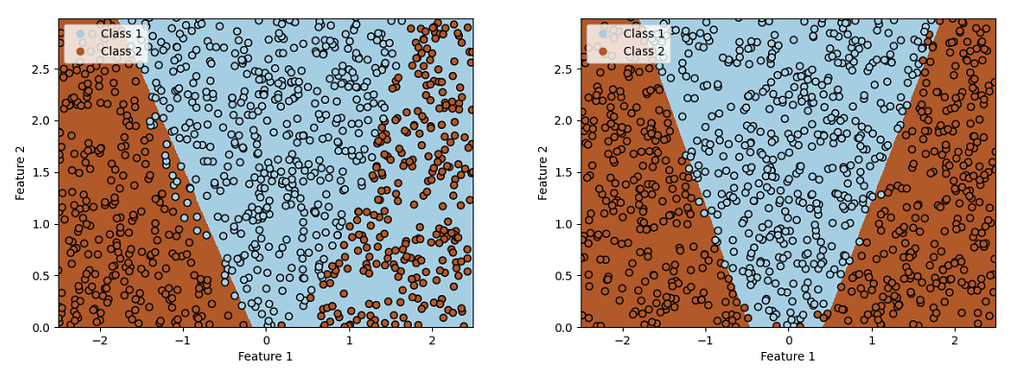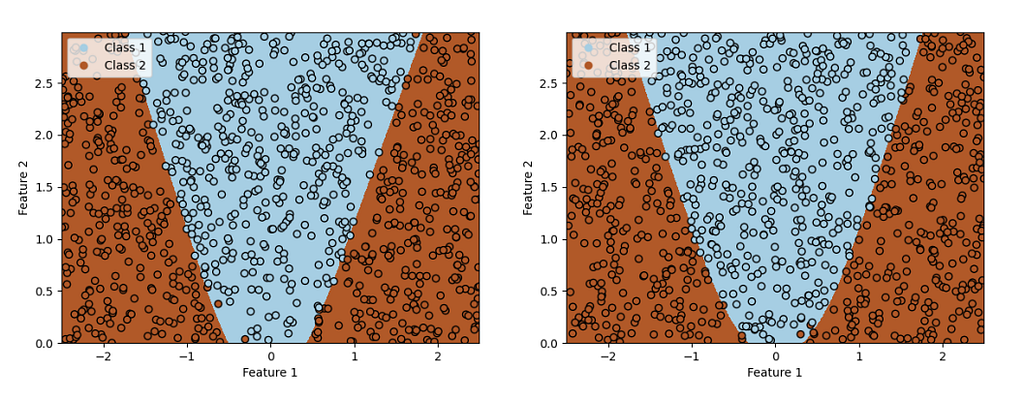#### A slightly different approach to understanding neural networks

Whenever I read a textbook about neural nets the introductions have always been quite similar: they mostly start with a motivation derived from biology. This has always bugged me a little bit. I am by no means a biologist but in my point of view there a quite some differences between biological and artificial neural networks:

1. Neural networks (usually) have a defined topology and learning takes place through the modification of weights. Natural neurons on the other hand constantly change their structure to learn.
2. Biological neurons have an “all-or-nothing”-policy: either a stimulus gets through or it doesn’t, whereas artificial neurons have smooth activation functions.
3. Biological neurons have a much higher fault and error tolerance.

So, because of these things it has never been really clear to me why the abstract mathematical representation of a biological concept works so well in practice. In this article I want to approach the topic from a different side, namely from statistics rather than biology.

### Logistic regression: Introduction

To approach neural nets we at first take a step back to “classical machine learning”, specifically logistic regression. Let us start with a simple binary classification problem, i.e. we want to distinguish between two different classes. We take a simple example (see picture below). Based on two features (arbitrarily named feature 1 and feature 2) we want to determine which class a given data point belongs to.

So, to formulate our problem a little bit more mathematically, we want a model to give us the probability that a data point belongs to class 1, given feature 1 and feature 2:

To make the writing a little bit simpler we will from now on denote our class as y and our features as x1, x2 = x, i.e. the above expressions becomes

Important: remember that we only have two classes, so if we have calculated the probability that a data point belongs to class 1 we can calculate the probability that the data point belongs to class 2 very easily:

Bayesian statistic gives us a rule on how to reformulate formula 1:

Marginalization allows us to rewrite Equation 1 as

Now we divide both the denominator and the numerator by P(y1|x) P(x) so that we get

Now we do one last step and rewrite equation 3once again.

We basically just replace the equation in the denominator with an exponential function. Remember that the natural logarithm and the exponential function cancel each other out.

The next step is really important because it shows why we call this classification technique logistic regression. What logistic regression does at its core is that we search for weights of our features so that they approximate our α:

In our case D is 2, because we just have two classes. w0 is a bias we also add. So, to achieve our task to distinguish between the two classes we need to learn the three weights w0, w1 and w2.

### Logistic regression: Application

I don’t want to go over the actual learning of these parameters because there are already a lot of great articles out there covering this topic. So we will now jump straight to the application of the model! Let us throw our logistic regression at the data above (image 1). After 500 training epochs we get the following result:

Whoah, that looks good! Our model can nearly perfectly discriminate between the two classes. Let’s try another dataset to see how the model performs.

Trained on the data in image 3 we get the following result:

Hm, this model does not perform well at all. It looks more like a random straight line drawn through the data. What is the reason for this? To get the answer, we have to look back at equation 5. What we see here is that the logistic regression multiplies the features with a weight and sums them all up. So what we have here is a linear model. Logistic regression can only learn to classify data that is linearly separable, or in layman’s terms, data that can clearly be divided by a straight line.The data we have here is obviously not linear separable as the border between the two classes has the shape of a parabola. But we can overcome this problem fairly easily. So far we have just fed two values in our model: feature 1 and feature 2. Now we just add a third feature, (features 1)². So we just square the value of feature 1. Trained again on the same data we get the result in image 5.

With this additional feature, our model fits the data nearly perfectly. But is this really a feasible solution? We want our model to be as general as possible so that we can fit as many different data distributions as possible. And there are a ton of non-linear functions. So if we want to perform the logistic regression well on many datasets apart from the squared value we will have to add the cube of our input features, the sine, the square root, and all kinds of other non-linear functions. We can construct a modified logistic regression where we don’t feed the features into the model directly. Instead of this, we define some kind of black box that takes the original features and applies various linear and non-linear transformations.

Instead of dealing with mathematical equations as we did before, let us illustrate the standard logistic regression (image 6) and our modified version (image 7) as graphs.

Both of these pictures look oddly similar to neural nets — because they are! Let that sink in for a moment: the standard logistic regression is nothing else than a neural net without a hidden layer. Our modified version is indeed a neural net with a hidden layer. With the major difference that in a real neural net we do not have to craft the functions in the hidden layer by hand as we did here.

### Classification with Neural Networks

Let us now compare the results we achieved with logistic regression with an actual neural net. First of all, we try a neural network without a hidden layer (image 8).

No surprise, the performance of this neural net is as bad as the standard logistic regression. Only when we start to add hidden layers with non-linear activation functions we can learn non-linear relations. So let’s try with a different number of hidden neurons.Image 9: Left: neural net with one hidden neuron. Right: neural net with two hidden neuronsImage 10: Left: neural net with 12 hidden neurons. Right: neural net with 50 hidden neurons

As we can see in the pictures above the net with just one hidden neuron performs pretty bad whereas two neurons already seem to be sufficient for a good classification. It looks like the net is able to find two straight decision boundaries on both sides. But the more hidden neurons we add, the smoother the boundary gets. This is especially visible in the bottom center.

Now let us take a look at the inner working of our net. The Keras library I used for this project makes it fairly easy to take a look at the output of hidden layers.

The outputs of the hidden layer with one and two neurons are not a big surprise. With one neuron we can detect one border but not the other, whereas with two neurons we can already achieve pretty good results. Looking at the outputs of the networks with 12 and 50 hidden neurons we can make two observation:

1. The outputs of quite a lot of neurons look very similar. So this might be a hint that we actually don’t need so many hidden neurons because a lot of them don’t add any additional information.
2. Instead of just the nearly linear and really sharp borders, we see in the first two networks we see quite some outputs where the output values are in a really narrow range and don’t form a sharp border. So these outputs probably help to make the overall classification more smooth.

Overall we can be happy with the results. Instead of having to come up with a good transformation of our input features like we did with the logistic regression, it took our net just a few hidden neurons and some seconds of training to come up with good transformations itself.

### Conclusion

I hope that this article gave you an interesting new view on neural networks and made it a little bit clearer where the idea of them comes from and why they work so well. So maybe next time you come across neural networks do not view them as just an abstract model of a biological neuron but of a non-linear feature transformer (hidden layer) combined with logistic regression (output layer) — a fairly easy concept that is not something magical and not “a black box that just works”!Biology ≠Technology was originally published in Towards Data Science on Medium, where people are continuing the conversation by highlighting and responding to this story.